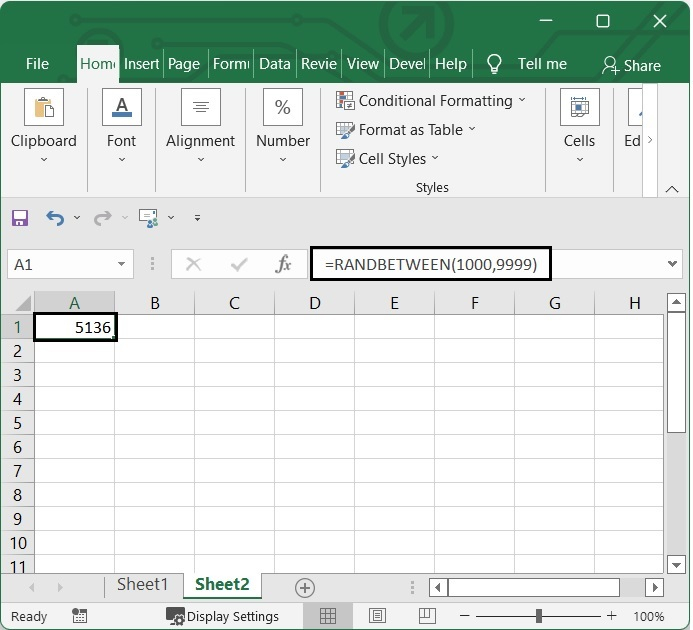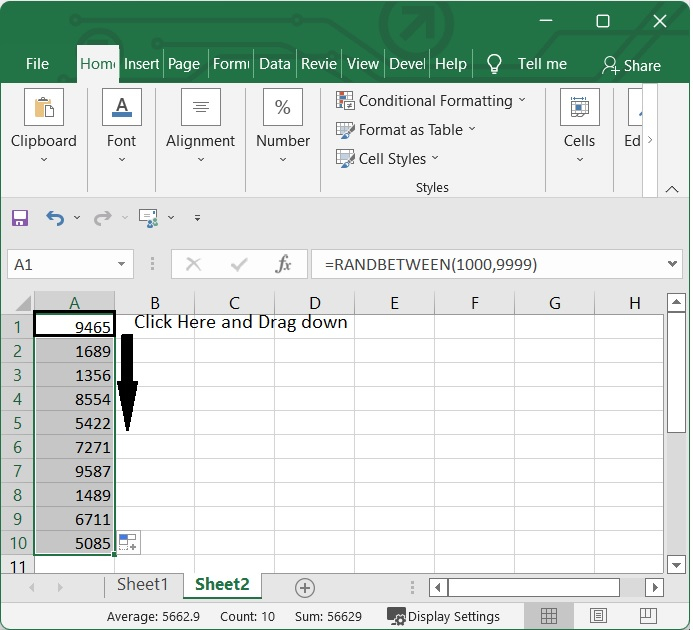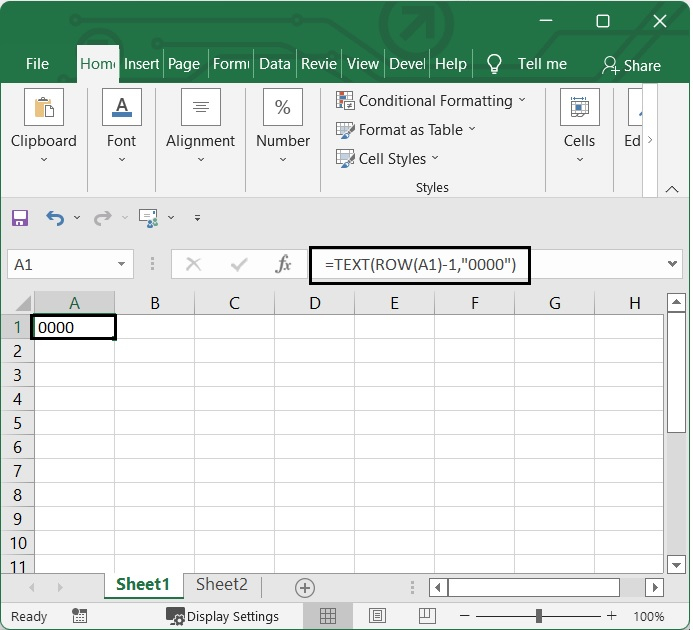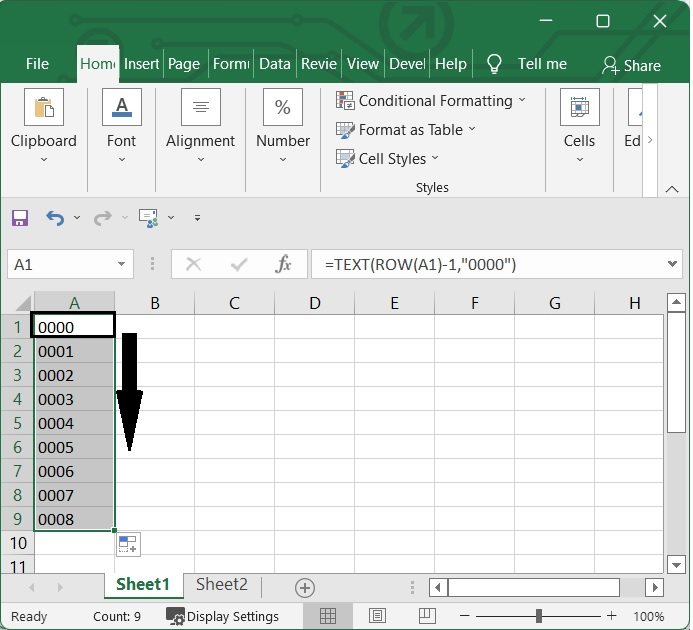# How to generate a list of all possible 4 digits combinations in Excel?

In certain circumstances, it may be necessary for us to make a list of all of the conceivable four-digit combinations of the numbers 0 to 9, which would require us to generate a list that includes the digits 0000, 0001, 0002, and 99999. For a speedy solution to the list problem in Excel. It is possible that we'll need to produce random numbers in Excel at some point. Numerous tasks, including the simulation and description of complex occurrences, the selection of random samples from larger data sets, and the generation of data encryption keys can all be accomplished with the help of random numbers.

Within the scope of this articles, we will explore a variety of approaches to the creation of an Excel-based random number generator with four digits.

## Generate four Digit Random Number using RANDBETWEEN Function

In Excel, the RANDBETWEEN function may be found in the Math and Trigonometry section of the function library. Excel's RANDBETWEEN function is responsible for producing a random value that falls between two specified values. Whenever a spreadsheet is opened or modified, RANDBETWEEN will produce a nearly new one. Let's use this function to produce some random integers with 4 digits.

Step 1

In an excel sheet, select one cell and add the following formula in it. In our case we have selected B1 and added the formula in it.

 =RANDBETWEEN(1000,9999)

After adding the formula, press enter. You will see a random number between 1000 to 9999 has been produced.

See the below given image.Step 2

To reflect in other cells, click on the ‘+’ sign appears on the lower right corner of the cell B1, which activates the autofill function and then drag down.

See the below given image.## Generate four Digit Random Number using TEXT Function

Excel's TEXT function takes a number and returns it in the format specified by the user as text. You can include formatted numbers within the body of text by making use of the TEXT function.

Step 1

In an excel sheet, select one cell and add the following formula in it. In our case we have selected A1 and added the formula in it.

 =TEXT(ROW(A1)-1,"0000")

After adding the formula, press enter. You will see a combination of 4 digit numbers has been produced.

See the below given image.Step 2

After that, pull the autofill handle to the bottom until all the possible combinations of 4 digits are listed.